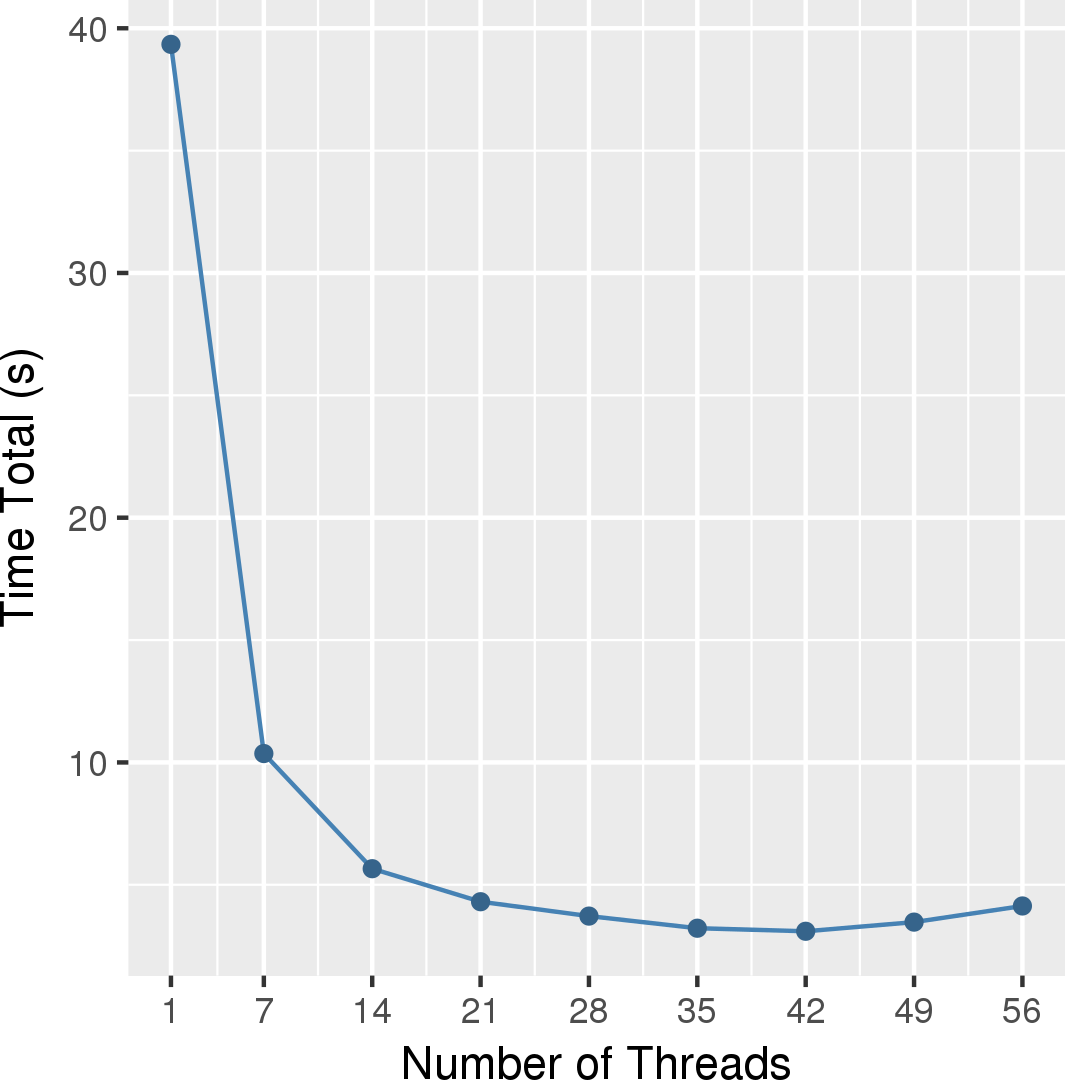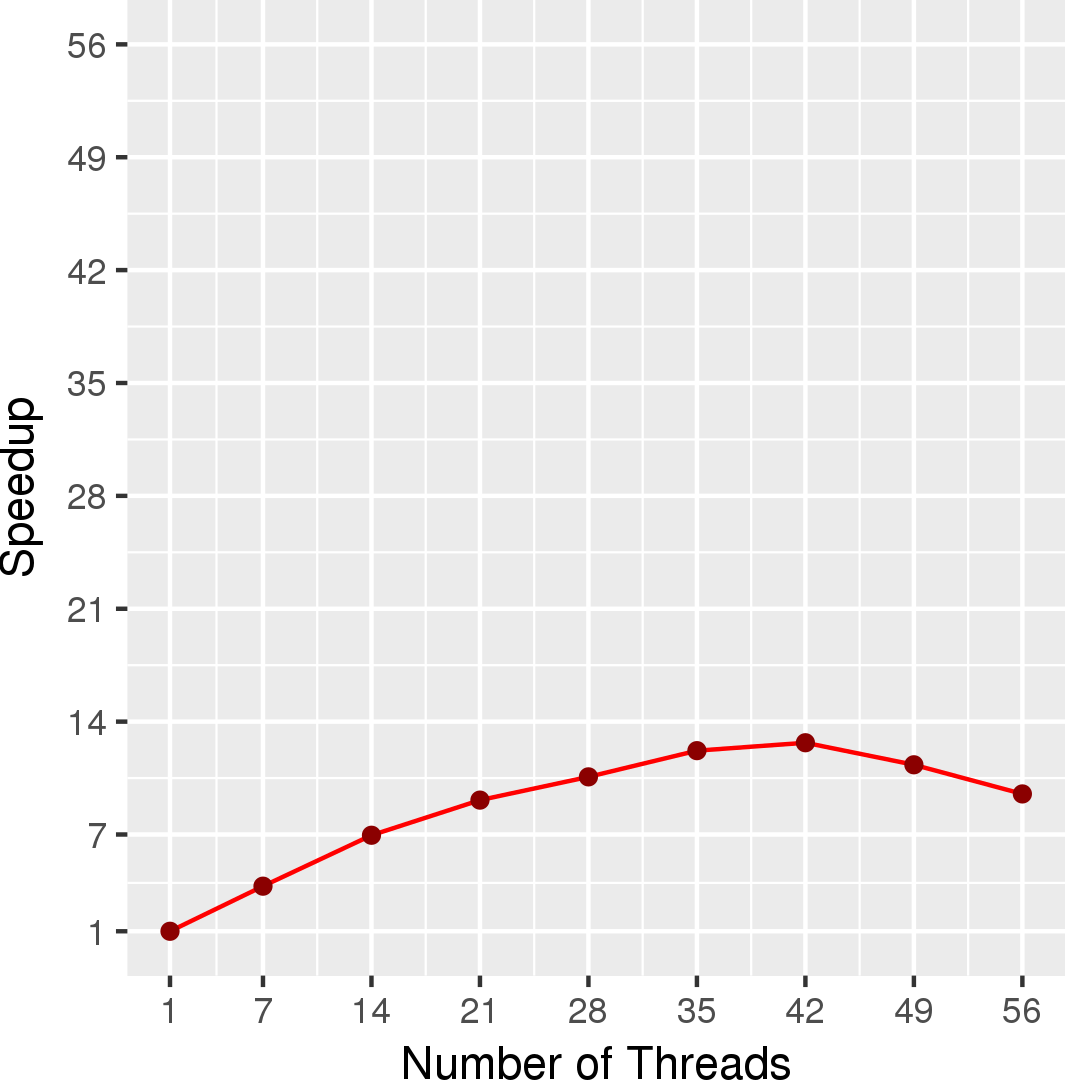# Maximum Cardinality Bipartite Matching

## Application Description

Given a graph G, a matching M is a subset of edges where no two edges share an endpoint. The cardinality |M| of M is the number of edges in M . Given an unweighted bipartite graph G = (V, E) V = (A, B), the maximum cardinality bipartite matching problem is to find a matching with maximum cardinality.

## Algorithm

Algorithms to solve this problem try to extend existing matchings by finding augmenting paths. When there are no more augmenting paths, the matching is a maximum matching.

The Ford-Fulkerson algorithm  visits each node in A trying to find an augmenting path at each. It has complexity O(|V||E|). The Alt-Blum-Mehlhorn-Paul (ABMP) algorithm  tries to find the shortest augmenting path first. After all the augmenting paths of less than a particular length are found or the number of unmatched nodes is reduced to a particular size, the algorithm applies the Ford-Fulkerson algorithm to complete the matching. The ABMP algorithm has complexity O(|E|sqrt(|V|)).

This benchmark implements the Alt-Blum-Mehlhorn-Paul algorithm.

 K. Mehlhorn and S. Naeher. LEDA: A Platform for Combinatorial and Geometric Computing. Cambridge University Press, 1999.

## Data Structures

There are two key data structures used in this algorithm:

Priority Scheduler
The ABMP algorithm uses priority on tasks to find the shortest augmenting paths first.
Directed Graph